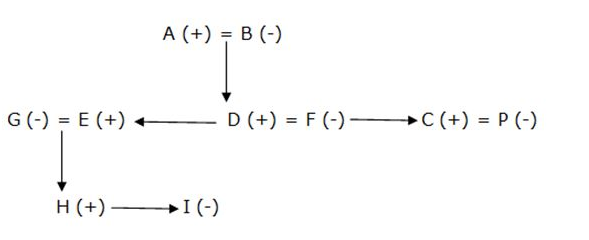# IBPS PO Prelims Reasoning Ability Questions 2019 (Day-13)

Dear Aspirants, Our IBPS Guide team is providing new series of Reasoning Questions for IBPS PO Prelims 2019 so the aspirants can practice it on a daily basis. These questions are framed by our skilled experts after understanding your needs thoroughly. Aspirants can practice these new series questions daily to familiarize with the exact exam pattern and make your preparation effective.

Check here for IBPS PO Prelims Mock Test 2019

[WpProQuiz 7144]

Directions (1-5): Study the following information carefully and answer the below questions.

Certain number of people sits at the circular table facing center.
Only three persons sit between I and S. Six people sit between J and R when counted from either left or right of R. Only two people sit between J and S. T sits third to the right of R. K sits seventh to the Right of M. K sits exactly between R and Q. R sits fifth to the left of S. P sits second to the left of M.R and Q are not an immediate neighbors. I doesn’t sit fifth to the right of P. Five people are sitting between S and Q.

1) How many seats between Q and J?(counted from left of J)?

a) 11

b) 14

c) 8

d) 17

e) None of these

2) What is the position of I with respect to K?

a) Second to the right

b) Third to the left

c) Second to the left

d) Third to the right

e) None of these

3) Four of the following five are alike in certain way and thus form a group as per the given arrangement. Which of the following does not belong to that group?

a) R-K

b) T-I

c) T-S

d) M-P

e) J-I

4) Which one of the following statements is correct?

a) Only one person sits between R and T

b) Q sits second left of K

c) T is an immediate neighbour of J and R

d) Only three persons sit between M and I

e) None of these

5) What is the total number of seats and persons in the table respectively?

a) 15, 10

b) 16, 8

c) 14, 9

d) 15, 9

e) 18, 9

Directions (6-7): Study the following information carefully and answer the below questions.

Point B is 8m north of Point A. Point C is 4m east of Point B. Point D is 6m north of point C. Point E is 10m West of point D. Point F is 6m east of point E. Point G is 6m east of point F.

6) What is the shortest distance between D and G?

a) 10m

b) 4m

c) 6m

d) 2m

e) None of these

7) Which of the following points are in the straight line?

a) C, G

b) B, F

c) A, D

d) E, B

e) None of these

Directions (8-10): Study the following information carefully and answer the below questions.

C is the son of A. E is the brother of D. D is the son of B. F is sister in law of E. I is the niece of D. H is the brother of I. G is the mother of H. A is the grandfather of I. C married to P. A has no daughters.

8) How is P related to B?

a) Daughter in law

b) Daughter

c) Grandson

d) Sister

e) None of these

9) How is H related to C?

a) Niece

b) Nephew

c) Son

d) Brother

e) None of these

10) How E’s daughter is related to P?

a) Can’t be determined

b) Grandson

c) Daughter

d) Sister

e) None of these

Directions (1-5):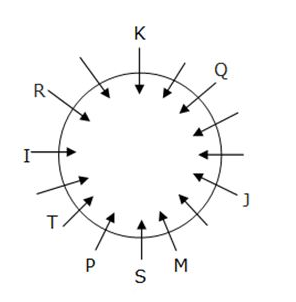Only three persons sit between I and S.

Five people are sitting between S and Q.

Only two people sits between J and S.

Six people sit between J and R when counted from either left or right of R.

R sits fifth to the left of S.

R and Q are not immediate neighbours.

Now we have 2 Cases.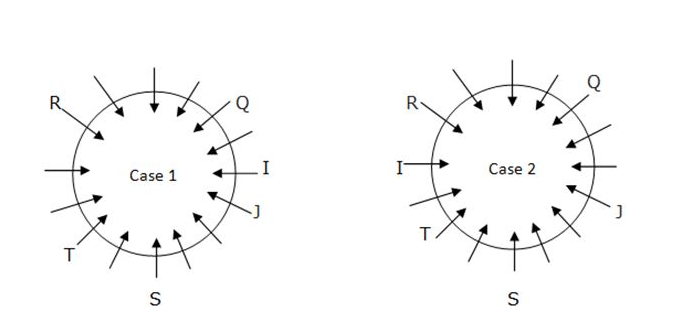K sits seventh to the Right of M.

K sits exactly between R and Q.

P sits second to the left of M.

I doesn’t sit fifth to the right of P.

So the final arrangement is.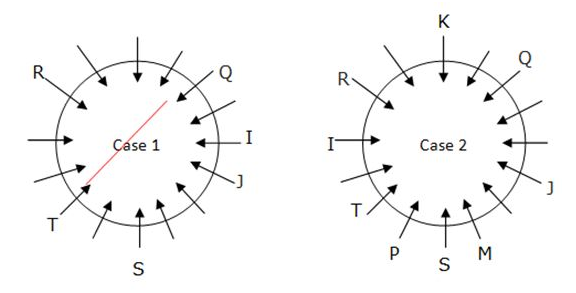Incase 1,K sits exactly between R and Q, this condition is not satisfied so rejected.

Directions (6-7):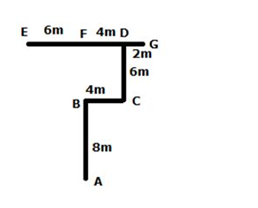Directions (8-10):